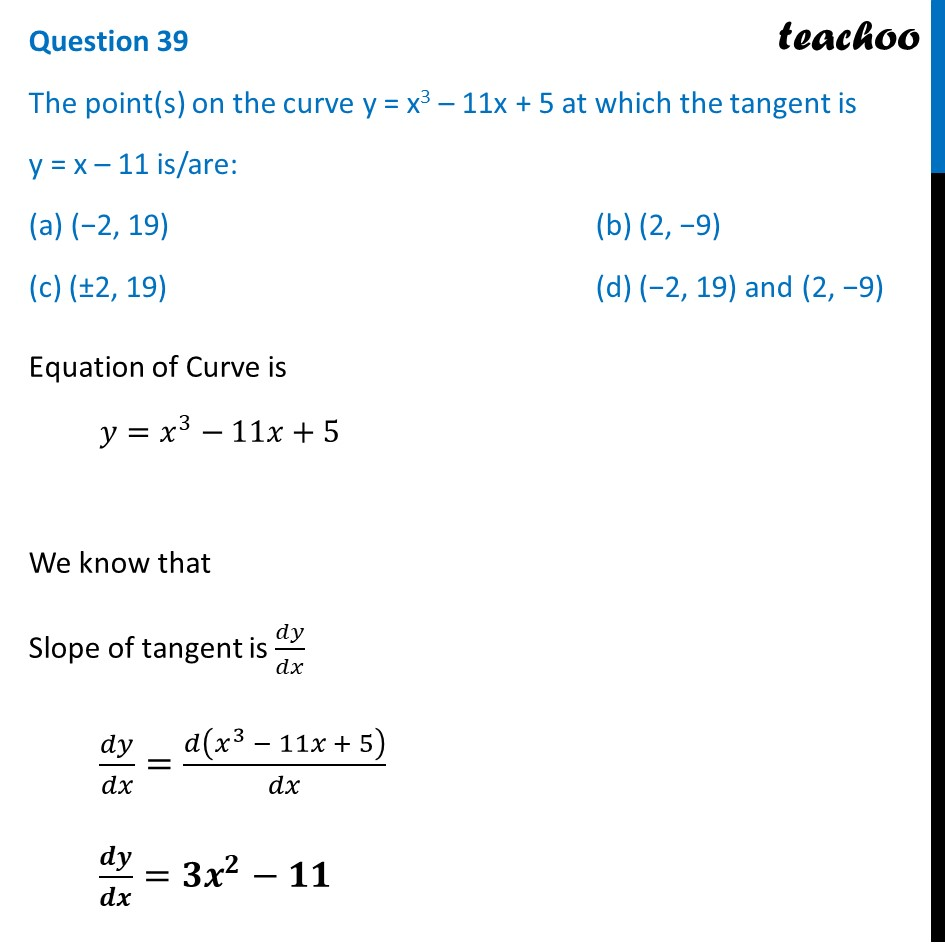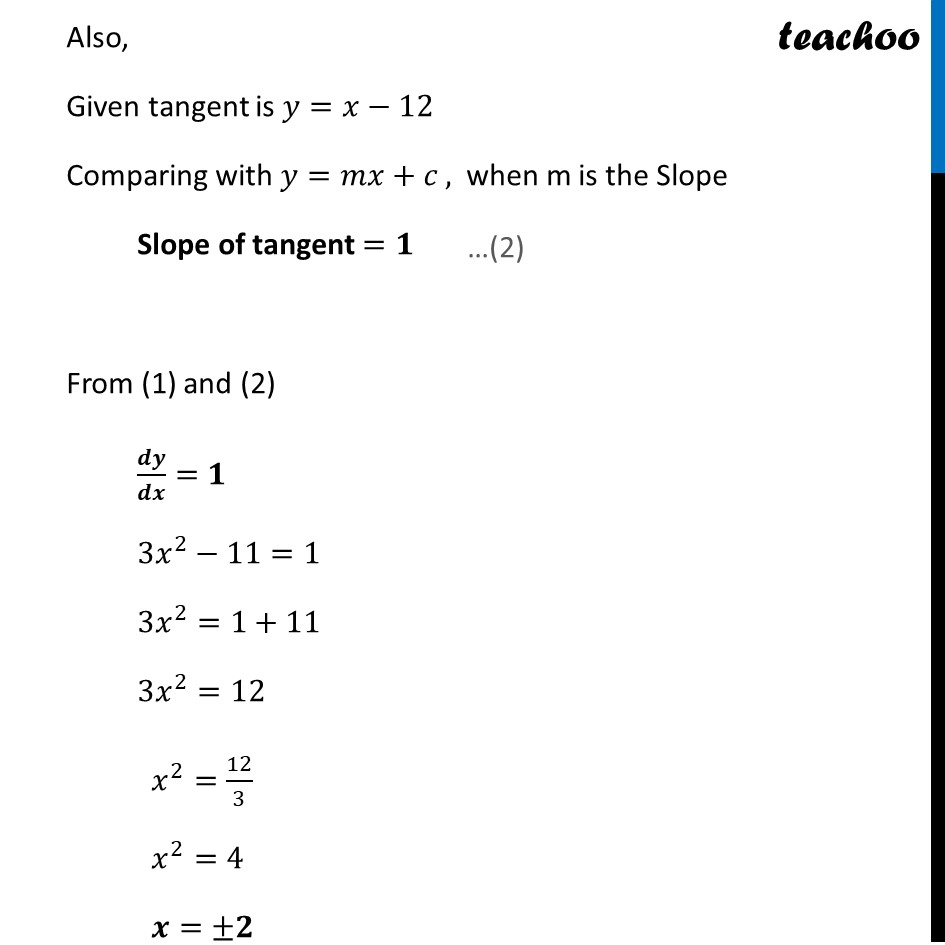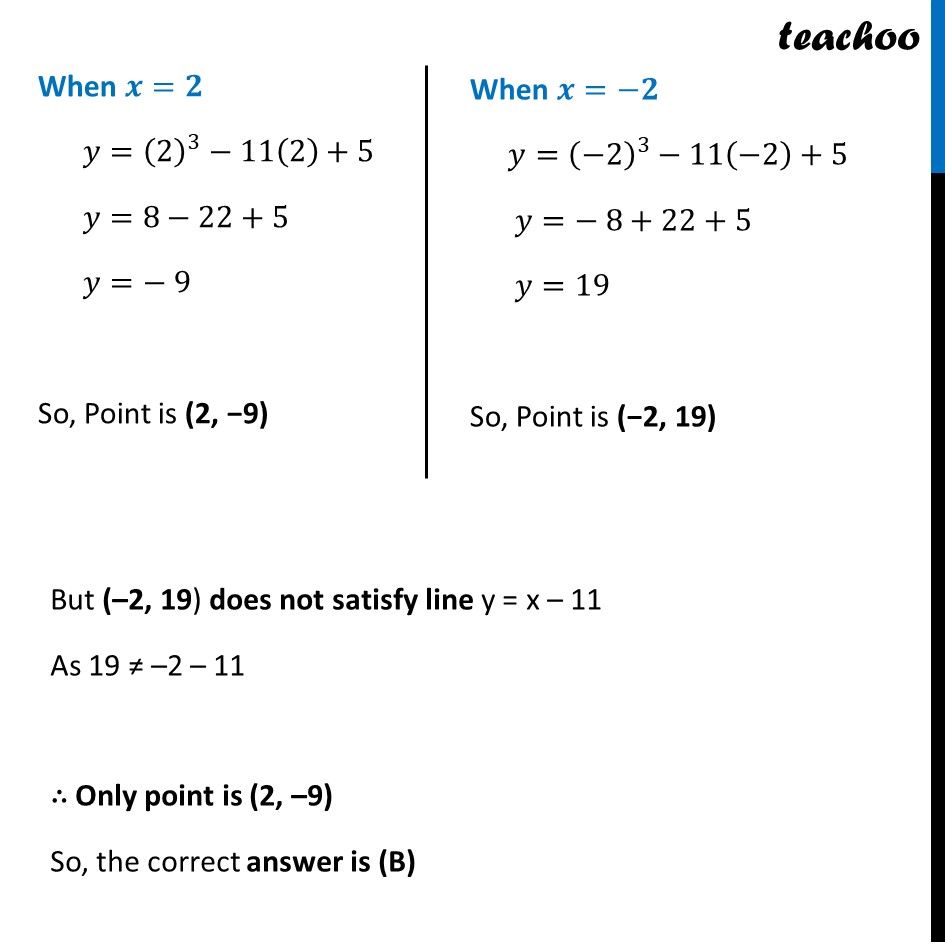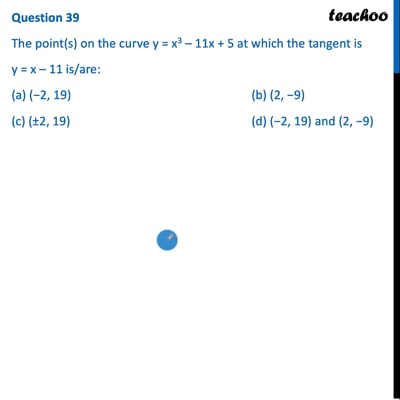CBSE Class 12 Sample Paper for 2022 Boards (MCQ Based - for Term 1)

Class 12
Solutions of Sample Papers and Past Year Papers - for Class 12 Boards

## (c) (±2, 19)       (d) (−2, 19) and (2, −9)

This question is inspired from Ex 6.3,9 - Chapter 6 Class 12 - Application of DerivativesThis video is only available for Teachoo black users

Introducing your new favourite teacher - Teachoo Black, at only ₹83 per month

### Transcript

Question 39 The point(s) on the curve y = x3 – 11x + 5 at which the tangent is y = x – 11 is/are: (a) (−2, 19) (b) (2, −9) (c) (±2, 19) (d) (−2, 19) and (2, −9) Equation of Curve is 𝑦=𝑥^3−11𝑥+5 We know that Slope of tangent is 𝑑𝑦/𝑑𝑥 𝑑𝑦/𝑑𝑥=𝑑(𝑥^3 − 11𝑥 + 5)/𝑑𝑥 𝒅𝒚/𝒅𝒙=〖𝟑𝒙〗^𝟐−𝟏𝟏 Also, Given tangent is 𝑦=𝑥−12 Comparing with 𝑦=𝑚𝑥+𝑐 , when m is the Slope Slope of tangent =𝟏 From (1) and (2) 𝒅𝒚/𝒅𝒙=𝟏 3𝑥^2−11=1 3𝑥^2=1+11 3𝑥^2=12 𝑥^2=12/3 𝑥^2=4 𝒙=±𝟐 When 𝒙=𝟐 𝑦=(2)^3−11(2)+5 𝑦=8−22+5 𝑦=− 9 So, Point is (2, −9) When 𝒙=−𝟐 𝑦=(−2)^3−11(−2)+5 𝑦=− 8+22+5 𝑦=19 So, Point is (−2, 19) But (–2, 19) does not satisfy line y = x – 11 As 19 ≠ –2 – 11 ∴ Only point is (2, –9) So, the correct answer is (B)